# Contents

require(IgGeneUsage)
require(rstan)
require(knitr)
require(ggplot2)
require(ggforce)
require(gridExtra)
require(ggrepel)
require(reshape2)

# 1 Introduction

Decoding the properties of immune repertoires is key in understanding the response of adaptive immunity to challenges such as viral infection. One important property is biases in immunoglobulin (Ig) gene usage between biological conditions (e.g. healthy vs tumor). Yet, most analyses for differential gene usage (DGU) are performed qualitatively, or with inadequate statistical methods. Here we introduce IgGeneUsage, a computational tool for DGU analysis.

# 2 Input

The main input of IgGeneUsage is a data.frame that has the following 4 columns:

1. sample_id: name of the repertoire (e.g. Patient-1)
2. condition: name of the condition to which each repertoire belongs (healthy, tumor_A, tumor_B, …)
3. gene_name: gene name (e.g. IGHV1-10 or family TRVB1)
4. gene_usage_count: numeric (count) of usage related in sample x gene x condition specified in columns 1-3

The sum of all gene usage counts (column 4) for a given repertoire is equal to the repertoire size (number of cells in the repertoire).

# 3 Model

IgGeneUsage transforms the provided input in the following way. Given $$R$$ repertoires, each having $$G$$ genes, IgGeneUsage generates a gene usage matrix $$Y^{R \times G}$$. Row sums in $$Y$$ define the total usage in each repertoire ($$N$$).

For the analysis of DGU between biological conditions, we designed the following Bayesian model ($$M$$) for zero-inflated beta-binomial regression. This model can fit over-dispersed gene usage data. The immune repertoire data is also not exhaustive, i.e. some Ig genes that are systematically rearranged at low probability might not be sampled, and certain Ig genes are not encoded (or dysfunctional) in some individuals. The zero-inflated component of our model accounts for this.

\begin{align} p(Y_{ijk} \mid M) = \begin{cases} \kappa_{j} + (1 - \kappa) \operatorname{BB}\left(0 \mid N_{ijk}, \theta_{ijk}, \phi \right), & \text{if Y_{ijk} = 0} \\ (1 - \kappa_{j}) \operatorname{BB}\left(Y_{ijk} \mid N_{ijk}, \theta_{ijk}, \phi \right), & \text{if Y_{ijk} > 0} \end{cases}\\ \theta_{ij}=\operatorname{logit^{-1}}\left(\alpha_{j}+\beta_{ijk}\right)\\ \beta_{ijk}\sim\operatorname{Normal}\left(\gamma_{jk},\sigma_{k}\right)\\ \gamma_{jk}\sim\operatorname{Normal}\left(0,\tau_{k}\right)\\ \alpha_{j}\sim\operatorname{Normal}\left(\mu_{\alpha},\sigma_{\alpha}\right)\\ \mu_{\alpha} \sim \operatorname{Normal}\left(0, 5\right)\\ \sigma_{k}, \tau_{k}, \sigma_{\alpha} \sim \operatorname{Cauchy^{+}}\left(0, 1\right) \\ \phi \sim \operatorname{Exponential}\left(\tau\right) \\ \tau \sim \operatorname{Gamma}\left(3, 0.1\right) \\ \kappa_{j} \sim \operatorname{Beta}\left({0.1, 1.0\right) \end{align}

Model $$M$$ legend:

• $$i$$ and $$j$$: index of different repertoires and genes, respectively
• $$\kappa$$: zero-inflation probability
• $$\theta$$: probability of gene usage
• $$\phi$$: dispersion
• $$\alpha$$: intercept/baseline gene usage
• $$\beta$$: slope/within-repertoire DGU coefficient
• $$\gamma$$, $$\gamma_{\sigma}$$: slope/gene-specific DGU coefficient; standard deviation
• $$\hat{\gamma}$$, $$\hat{\gamma}_{\sigma}$$: mean and standard deviation of the population of gene-specific DGU coefficients
• $$\hat{\alpha}$$, $$\hat{\alpha}_{\sigma}$$: mean and standard deviation of the population of gene-specific baseline usages
• $$\operatorname{BB}$$: beta-binomial probability mass function (pmf)
• $$\operatorname{Normal}$$: normal probability density function (pdf)
• $$\operatorname{Cauchy^{+}}$$: half-Cauchy pdf
• $$\operatorname{Exponential}$$: exponential pdf
• $$\operatorname{Gamma}$$: gamma pdf
• $$\operatorname{Beta}$$: beta pdf
• $$\operatorname{logit^{-1}}$$: inverse logistic function

In the output of IgGeneUsage, we report the mean effect size (es or $$\gamma$$) and its 95% highest density interval (HDI). Genes with $$\gamma \neq 0$$ (e.g. if 95% HDI of $$\gamma$$ excludes 0) are most likely to experience differential usage. Additionally, we report the probability of differential gene usage ($$\pi$$): \begin{align} \pi = 2 \cdot \max\left(\int_{\gamma = -\infty}^{0} p(\gamma)\mathrm{d}\gamma, \int_{\gamma = 0}^{\infty} p(\gamma)\mathrm{d}\gamma\right) - 1 \end{align} with $$\pi = 1$$ for genes with strong differential usage, and $$\pi = 0$$ for genes with negligible differential gene usage. Both metrics are computed based on the posterior distribution of $$\gamma$$, and are thus related.

# 4 Case Study A

IgGeneUsage has a couple of built-in Ig gene usage datasets. Some were obtained from studies and others were simulated.

Lets look into the simulated dataset d_zibb_2. This dataset was generated by a zero-inflated beta-binomial (ZIBB) model, and IgGeneUsage was designed to fit ZIBB-distributed data.

data("d_zibb_2", package = "IgGeneUsage")
knitr::kable(head(d_zibb_2))
sample_id condition gene_name gene_usage_count
S1 C1 G1 244
S1 C1 G2 193
S1 C1 G3 139
S1 C1 G4 39
S1 C1 G5 440
S1 C1 G6 15

We can also visualize d_zibb_2 with ggplot:

ggplot(data = d_zibb_2)+
geom_point(aes(x = gene_name, y = gene_usage_count, col = condition),
position = position_dodge(width = .7), shape = 21)+
theme_bw(base_size = 11)+
ylab(label = "Gene usage [count]")+
xlab(label = '')+
theme(legend.position = "top")+
theme(axis.text.x = element_text(angle = 90, hjust = 1, vjust = 0.4))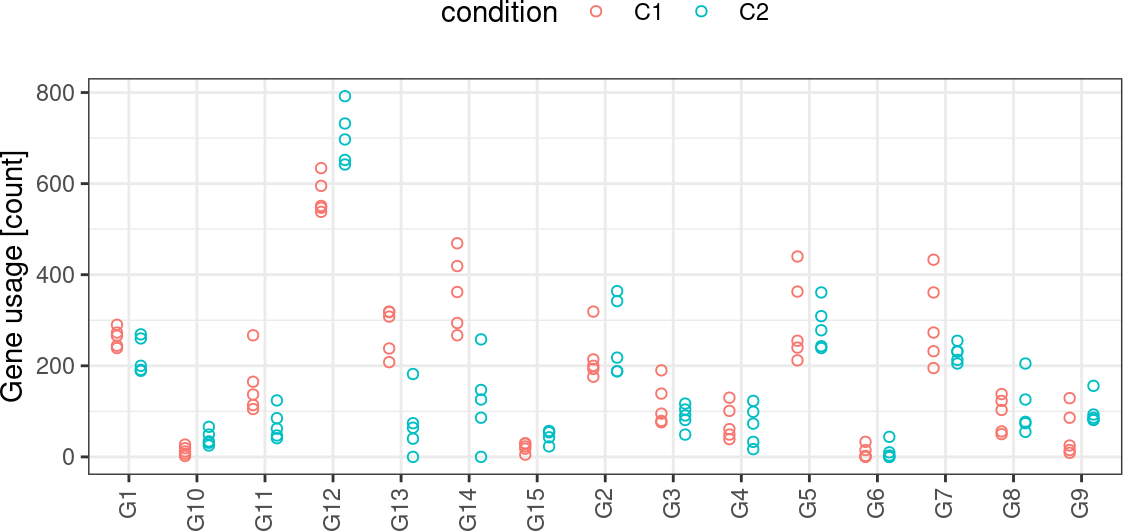## 4.1 DGU analysis

As main input IgGeneUsage uses a data.frame formatted as d_zibb_2. Other input parameters allow you to configure specific settings of the rstan sampler.

In this example we analyze d_zibb_2 with 2 MCMC chains, 1500 iterations each including 500 warm-ups using a single CPU core (Hint: for parallel chain execution set parameter mcmc_cores = 2). We report for each model parameter its mean and 95% highest density interval (HDIs).

Important remark: you should run DGU analyses using default IgGeneUsage parameters. If warnings or errors are reported with regard to the MCMC sampling, please consult the Stan manual and adjust the inputs accordingly. If the warnings persist, please submit an issue with a reproducible script at the Bioconductor support site or on Github.

M <- DGU(ud = d_zibb_2, # input data
mcmc_warmup = 500, # how many MCMC warm-ups per chain (default: 500)
mcmc_steps = 1500, # how many MCMC steps per chain (default: 1,500)
mcmc_chains = 2, # how many MCMC chain to run (default: 4)
mcmc_cores = 1, # how many PC cores to use? (e.g. parallel chains)
hdi_lvl = 0.95, # highest density interval level (de fault: 0.95)
adapt_delta = 0.8, # MCMC target acceptance rate (default: 0.95)
max_treedepth = 10) # tree depth evaluated at each step (default: 12)
FALSE
FALSE SAMPLING FOR MODEL 'dgu' NOW (CHAIN 1).
FALSE Chain 1:
FALSE Chain 1: Gradient evaluation took 0.000252 seconds
FALSE Chain 1: 1000 transitions using 10 leapfrog steps per transition would take 2.52 seconds.
FALSE Chain 1:
FALSE Chain 1:
FALSE Chain 1: Iteration:    1 / 1500 [  0%]  (Warmup)
FALSE Chain 1: Iteration:  150 / 1500 [ 10%]  (Warmup)
FALSE Chain 1: Iteration:  300 / 1500 [ 20%]  (Warmup)
FALSE Chain 1: Iteration:  450 / 1500 [ 30%]  (Warmup)
FALSE Chain 1: Iteration:  501 / 1500 [ 33%]  (Sampling)
FALSE Chain 1: Iteration:  650 / 1500 [ 43%]  (Sampling)
FALSE Chain 1: Iteration:  800 / 1500 [ 53%]  (Sampling)
FALSE Chain 1: Iteration:  950 / 1500 [ 63%]  (Sampling)
FALSE Chain 1: Iteration: 1100 / 1500 [ 73%]  (Sampling)
FALSE Chain 1: Iteration: 1250 / 1500 [ 83%]  (Sampling)
FALSE Chain 1: Iteration: 1400 / 1500 [ 93%]  (Sampling)
FALSE Chain 1: Iteration: 1500 / 1500 [100%]  (Sampling)
FALSE Chain 1:
FALSE Chain 1:  Elapsed Time: 10.767 seconds (Warm-up)
FALSE Chain 1:                9.631 seconds (Sampling)
FALSE Chain 1:                20.398 seconds (Total)
FALSE Chain 1:
FALSE
FALSE SAMPLING FOR MODEL 'dgu' NOW (CHAIN 2).
FALSE Chain 2:
FALSE Chain 2: Gradient evaluation took 0.000156 seconds
FALSE Chain 2: 1000 transitions using 10 leapfrog steps per transition would take 1.56 seconds.
FALSE Chain 2:
FALSE Chain 2:
FALSE Chain 2: Iteration:    1 / 1500 [  0%]  (Warmup)
FALSE Chain 2: Iteration:  150 / 1500 [ 10%]  (Warmup)
FALSE Chain 2: Iteration:  300 / 1500 [ 20%]  (Warmup)
FALSE Chain 2: Iteration:  450 / 1500 [ 30%]  (Warmup)
FALSE Chain 2: Iteration:  501 / 1500 [ 33%]  (Sampling)
FALSE Chain 2: Iteration:  650 / 1500 [ 43%]  (Sampling)
FALSE Chain 2: Iteration:  800 / 1500 [ 53%]  (Sampling)
FALSE Chain 2: Iteration:  950 / 1500 [ 63%]  (Sampling)
FALSE Chain 2: Iteration: 1100 / 1500 [ 73%]  (Sampling)
FALSE Chain 2: Iteration: 1250 / 1500 [ 83%]  (Sampling)
FALSE Chain 2: Iteration: 1400 / 1500 [ 93%]  (Sampling)
FALSE Chain 2: Iteration: 1500 / 1500 [100%]  (Sampling)
FALSE Chain 2:
FALSE Chain 2:  Elapsed Time: 10.564 seconds (Warm-up)
FALSE Chain 2:                9.641 seconds (Sampling)
FALSE Chain 2:                20.205 seconds (Total)
FALSE Chain 2:

## 4.2 Output format

The following objects are provided as part of the output of DGU:

• dgu_summary (main results of IgGeneUsage): quantitative DGU summary
• gu_summary: quantitative summary of the gene usage (GU) of each gene in the different conditions
• glm: rstan (‘stanfit’) object of the fitted model $$\rightarrow$$ used for model checks (see section ‘Model checking’)
• ppc: posterior predictive checks data (see section ‘Model checking’)
summary(M)
FALSE             Length Class      Mode
FALSE dgu_summary  9     data.frame list
FALSE gu_summary   8     data.frame list
FALSE glm          1     stanfit    S4
FALSE ppc          2     -none-     list
FALSE ud          10     -none-     list

## 4.3 Model checking

• Check your model fit. For this, you can use the object glm.

• Minimal checklist of successful MCMC sampling:
• no divergences
• no excessive warnings from rstan
• Rhat < 1.05
• high Neff
• Minimal checklist for valid model:
• posterior predictive checks (PPCs): is model consistent with reality, i.e. is there overlap between simulated and observed data?
• leave-one-out analysis

### 4.3.1 MCMC sampling

• divergences, tree-depth, energy
• none found
rstan::check_hmc_diagnostics(M$glm) FALSE FALSE Divergences: FALSE FALSE Tree depth: FALSE FALSE Energy: • rhat < 1.03 and n_eff > 0 gridExtra::grid.arrange(rstan::stan_rhat(object = M$glm),
rstan::stan_ess(object = M$glm), nrow = 1)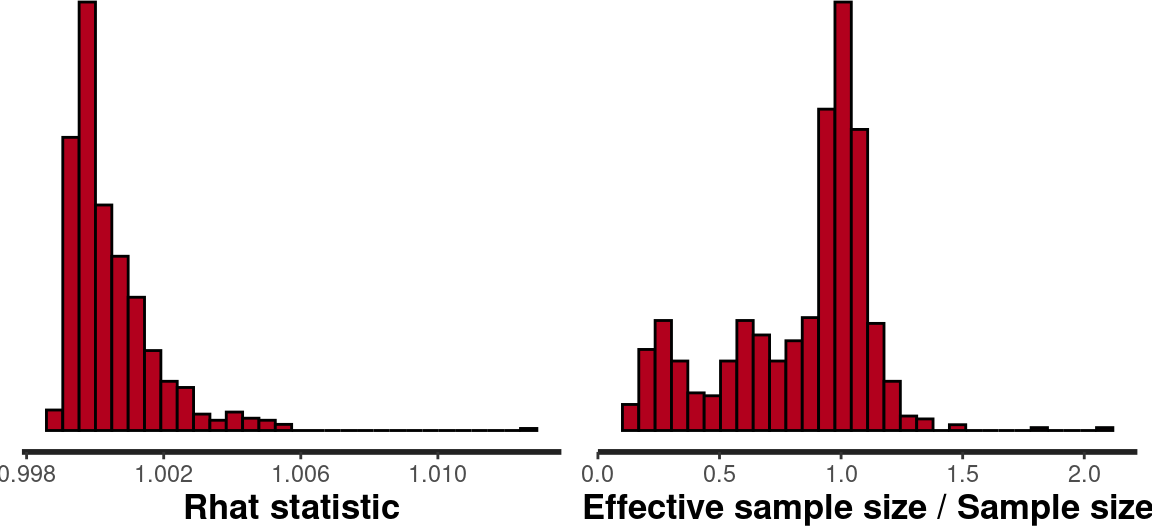## 4.4 PPC: posterior predictive checks ### 4.4.1 PPCs: repertoire-specific The model used by IgGeneUsage is generative, i.e. with the model we can generate usage of each Ig gene in a given repertoire (y-axis). Error bars show 95% HDI of mean posterior prediction. The predictions can be compared with the observed data (x-axis). For points near the diagonal $$\rightarrow$$ accurate prediction. ggplot(data = M$ppc$ppc_rep)+ facet_wrap(facets = ~sample_id, ncol = 5)+ geom_abline(intercept = 0, slope = 1, linetype = "dashed", col = "darkgray")+ geom_errorbar(aes(x = observed_count, y = ppc_mean_count, ymin = ppc_L_count, ymax = ppc_H_count), col = "darkgray")+ geom_point(aes(x = observed_count, y = ppc_mean_count), size = 1)+ theme_bw(base_size = 11)+ theme(legend.position = "top")+ xlab(label = "Observed usage [counts]")+ ylab(label = "PPC usage [counts]")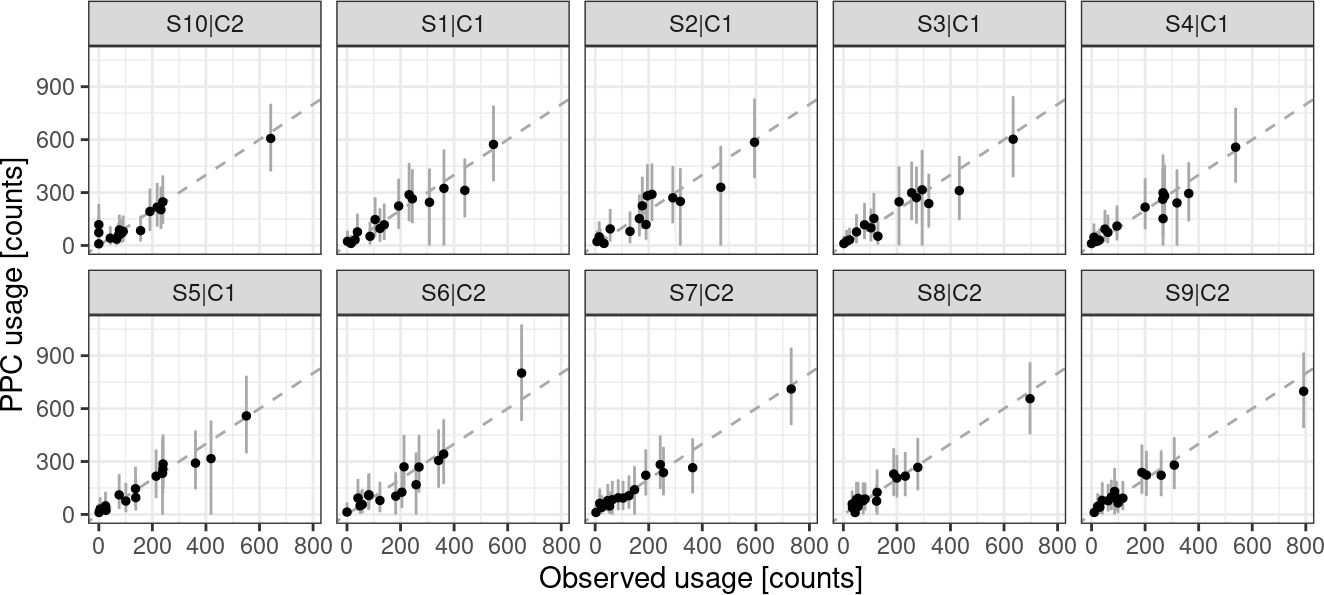### 4.4.2 PPCs: overall Prediction of generalized gene usage within a biological condition is also possible. We show the predictions (y-axis) of the model, and compare them against the observed mean usage (x-axis). If the points are near the diagonal $$\rightarrow$$ accurate prediction. Errors are 95% HDIs of the mean. ggplot(data = M$ppc$ppc_condition)+ geom_errorbar(aes(x = gene_name, ymin = ppc_L_prop*100, ymax = ppc_H_prop*100, col = condition), position = position_dodge(width = 0.65), width = 0.1)+ geom_point(aes(x = gene_name, y = ppc_mean_prop*100,col = condition), position = position_dodge(width = 0.65))+ theme_bw(base_size = 11)+ theme(legend.position = "top")+ xlab(label = "Observed usage [%]")+ ylab(label = "PPC usage [%]")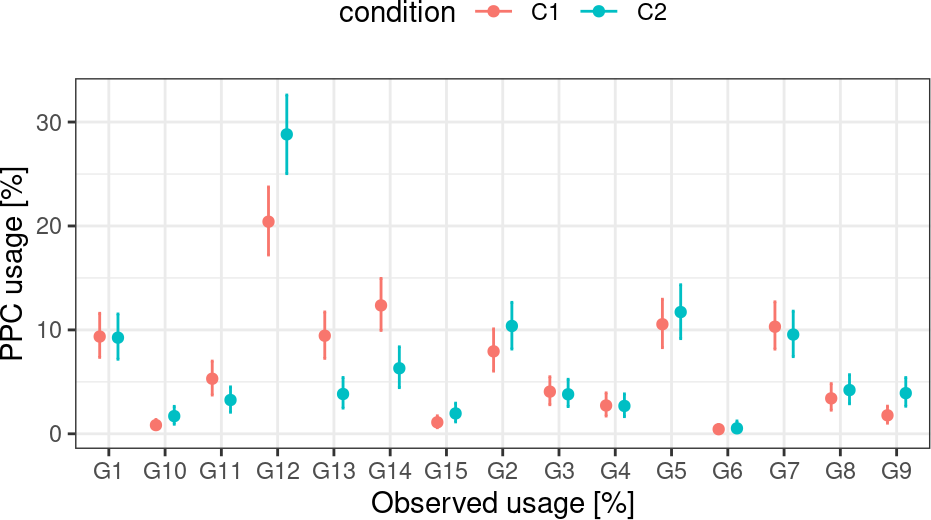## 4.5 Results Each row of glm_summary summarizes the degree of DGU observed for specific Igs. Two metrics are reported: • es (also referred to as $$\gamma$$): effect size on DGU, where contrast gives the direction of the effect (e.g. tumor - healthy or healthy - tumor) • pmax (also referred to as $$\pi$$): probability of DGU (parameter $$\pi$$ from model $$M$$) For es we also have the mean, median standard error (se), standard deviation (sd), L (low bound of 95% HDI), H (high bound of 95% HDI) kable(x = head(M$dgu_summary), row.names = FALSE, digits = 3)
es_mean es_mean_se es_sd es_median es_L es_H contrast gene_name pmax
0.013 0.004 0.181 0.009 -0.341 0.364 C1-vs-C2 G1 0.037
-0.732 0.009 0.380 -0.716 -1.474 -0.033 C1-vs-C2 G10 0.957
0.516 0.005 0.243 0.521 0.047 1.006 C1-vs-C2 G11 0.966
-0.457 0.003 0.135 -0.459 -0.722 -0.191 C1-vs-C2 G12 1.000
0.975 0.005 0.251 0.968 0.510 1.495 C1-vs-C2 G13 1.000
0.746 0.004 0.203 0.743 0.353 1.144 C1-vs-C2 G14 1.000

### 4.5.1 DGU: differential gene usage

We know that the values of \gamma and \pi are related to each other. Lets visualize them for all genes (shown as a point). Names are shown for genes associated with $$\pi \geq 0.9$$. Dashed horizontal line represents null-effect ($$\gamma = 0$$).

Notice that the gene with $$\pi \approx 1$$ also has an effect size whose 95% HDI (error bar) does not overlap the null-effect. The genes with high degree of differential usage are easy to detect with this figure.

# format data
stats <- M$dgu_summary stats <- stats[order(abs(stats$es_mean), decreasing = FALSE), ]
stats$gene_fac <- factor(x = stats$gene_name, levels = unique(stats$gene_name)) ggplot(data = stats)+ geom_hline(yintercept = 0, linetype = "dashed", col = "gray")+ geom_errorbar(aes(x = pmax, y = es_mean, ymin = es_L, ymax = es_H), col = "darkgray")+ geom_point(aes(x = pmax, y = es_mean, col = contrast))+ geom_text_repel(data = stats[stats$pmax >= 0.9, ],
aes(x = pmax, y = es_mean, label = gene_fac),
min.segment.length = 0, size = 2.75)+
theme_bw(base_size = 11)+
theme(legend.position = "top")+
xlab(label = expression(pi))+
xlim(c(0, 1))+
ylab(expression(gamma))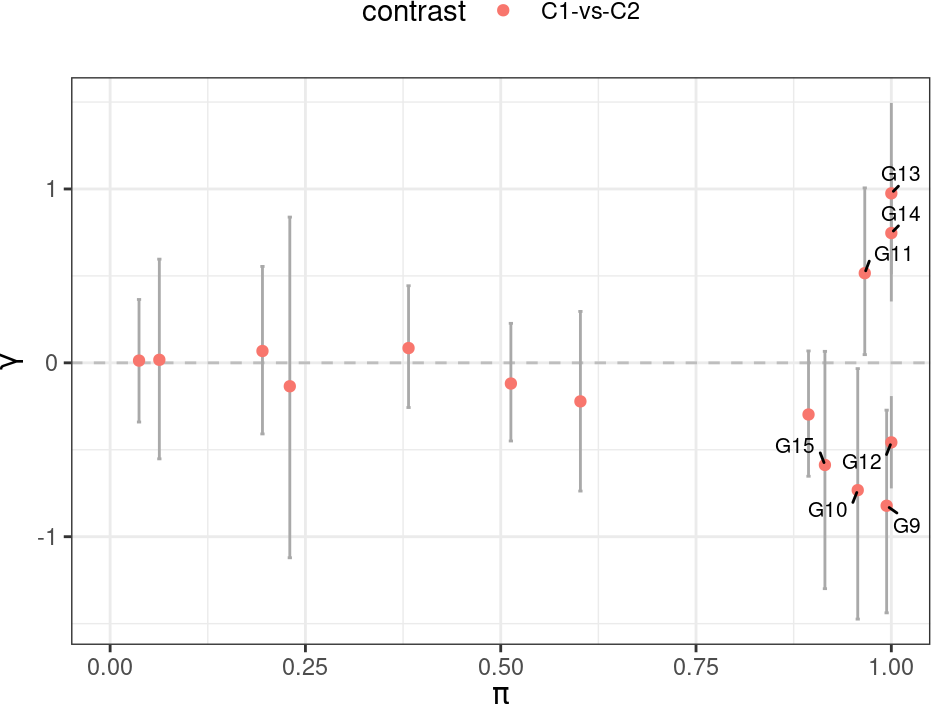### 4.5.2 Promising hits

Lets visualize the observed data of the genes with high probability of differential gene usage ($$\pi \geq 0.9$$). Here we show the gene usage in %.

promising_genes <- stats$gene_name[stats$pmax >= 0.9]

ppc_gene <- M$ppc$ppc_condition
ppc_gene <- ppc_gene[ppc_gene$gene_name %in% promising_genes, ] ppc_rep <- M$ppc$ppc_rep ppc_rep <- ppc_rep[ppc_rep$gene_name %in% promising_genes, ]

ggplot()+
geom_point(data = ppc_rep,
aes(x = gene_name, y = observed_prop*100, col = condition),
size = 1, fill = "black",
position = position_jitterdodge(jitter.width = 0.1,
jitter.height = 0,
dodge.width = 0.35))+
geom_errorbar(data = ppc_gene,
aes(x = gene_name, ymin = ppc_L_prop*100,
ymax = ppc_H_prop*100, group = condition),
position = position_dodge(width = 0.35), width = 0.15)+
theme_bw(base_size = 11)+
theme(legend.position = "top")+
ylab(label = "PPC usage [%]")+
xlab(label = '')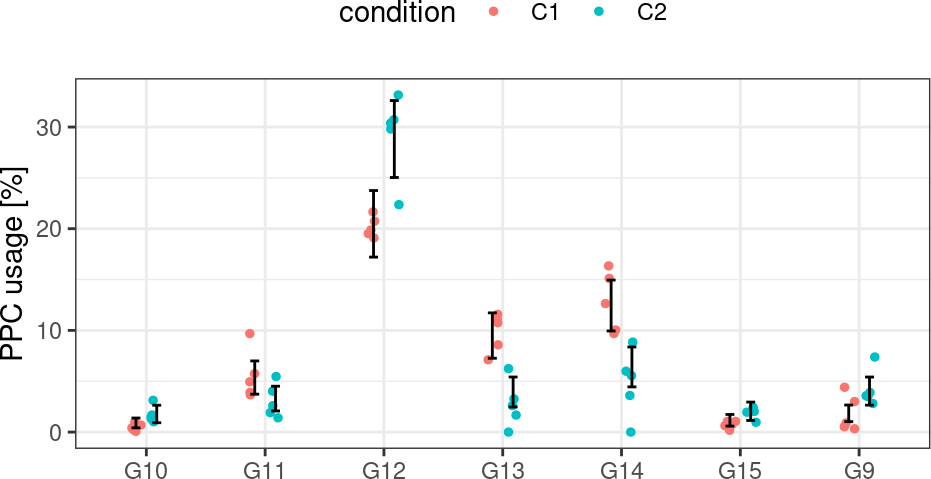### 4.5.3 Promising hits [count]

Lets also visualize the predicted gene usage counts in each repertoire.

ggplot()+
geom_point(data = ppc_rep,
aes(x = gene_name, y = observed_count, col = condition),
size = 1, fill = "black",
position = position_jitterdodge(jitter.width = 0.1,
jitter.height = 0,
dodge.width = 0.5))+
theme_bw(base_size = 11)+
theme(legend.position = "top")+
ylab(label = "PPC usage [count]")+
xlab(label = '')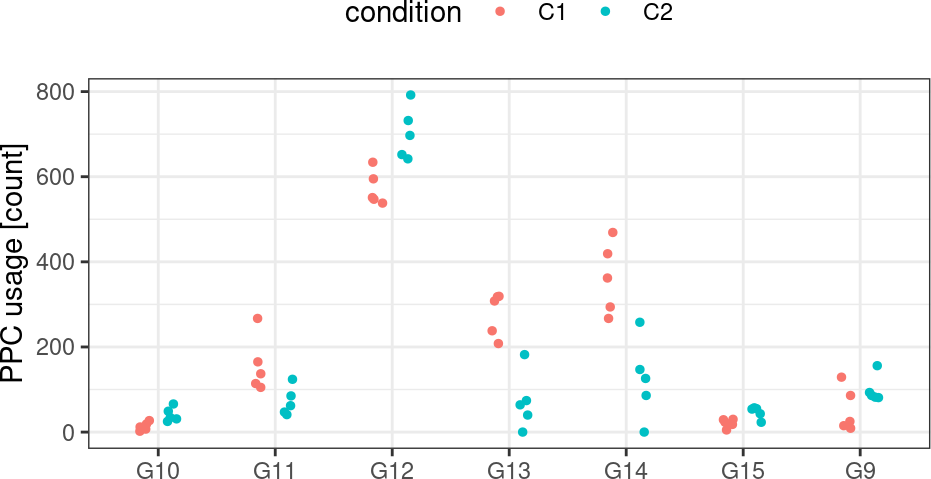## 4.6 GU: gene usage summary

IgGeneUsage also reports the inferred gene usage (GU) probability of individual genes in each condition. For a given gene we report its mean GU (prob_mean) and the 95% (for instance) HDI (prob_L and prob_H).

ggplot(data = M\$gu_summary)+
geom_hline(yintercept = 0, linetype = "dashed", col = "gray")+
geom_errorbar(aes(x = gene_name, y = prob_mean, ymin = prob_L,
ymax = prob_H, col = condition),
width = 0.1, position = position_dodge(width = 0.4))+
geom_point(aes(x = gene_name, y = prob_mean, col = condition), size = 1,
position = position_dodge(width = 0.4))+
theme_bw(base_size = 11)+
theme(legend.position = "top")+
ylab(label = "GU [probability]")